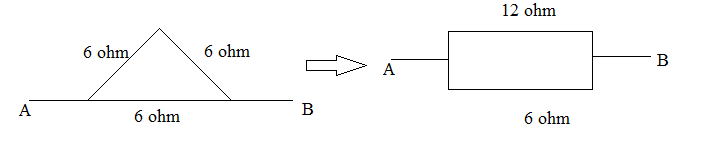A uniform metallic wire of resistance 18 ohm is bent to form an equilateral triangle. The resistance across any two vertices of the triangle is (in ohm).

a. 8 ohm

b. 12 ohm

c. 4 ohm

d. 2 ohmLet Req be resistance between any two vertex.

1/Req = (1/12)+(1/6)

So Req = 4 ohm(0)(0)